# MATLAB image display – truecolor and indexed images

### Contents

#### MATLAB image display - truecolor and indexed images

Last time I posed this question: How does MATLAB associate the value of particular array elements with a color displayed on the screen? Let's start by exploring MATLAB's two basic pixel-color display models:

• 3-D array element values specify pixel colors directly
• 2-D matrix element values specify pixel colors indirectly, through the figure or axes colormap

#### Truecolor images

I'll start by constructing a simple image containing just six pixels: red, green, blue, cyan, magenta, and yellow.

plane_1 = [1 0 0; 0 1 1];
plane_2 = [0 1 0; 1 0 1];
plane_3 = [0 0 1; 1 1 0];

rgb = cat(3,plane_1,plane_2,plane_3);
im = imshow(rgb,'InitialMagnification','fit');
title('Truecolor image with six pixels')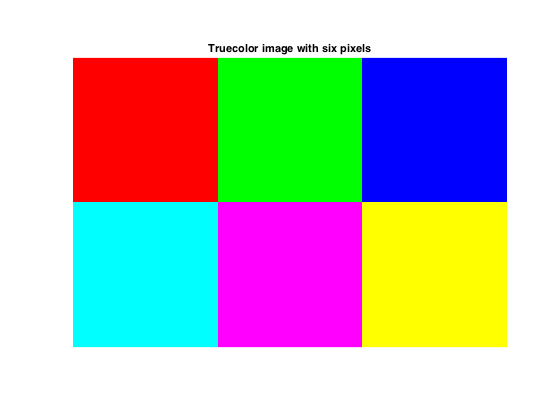So, what's going on here? Look first at the size of rgb:

size(rgb)

ans =

2     3     3



The first two numbers in the size of rgb tell you the number of rows and columns of pixels. There are two rows and three columns.

The size of rgb along the third dimension is 3. That's because we are using 3 different values to specify a pixel color. Here are the three values for the magenta color, which is row 2, column 2:

rgb(2,2,:)

ans(:,:,1) =

1

ans(:,:,2) =

0

ans(:,:,3) =

1



The three numbers represent an additive mix of red, green and blue light. The value 1 corresponds to full-intensity light, whereas the value 0 corresponds to no light. So the magenta color above is a mix of full-intensity red light, no green light, and full-intensity blue light. Similarly, here are the three values for the yellow pixel:

rgb(2,3,:)

ans(:,:,1) =

1

ans(:,:,2) =

1

ans(:,:,3) =

0



The yellow color is a mix of no red light, full-intensity green light, and full-intensity blue light.

This type of image, in which each pixel color is represented by three values, is often called a truecolor image.

Now let's peek at the Graphics image object, which was returned above by the call to imshow:

im

im =

Image with properties:

CData: [2x3x3 double]
CDataMapping: 'direct'


The object display shows the most commonly used properties of the image object, CData and CDataMapping. (I won't be talking further about CDataMapping today. We'll come back to it in one of the next couple of blog posts.)

CData, which stands for color data, contains the 2-by-3-by-3 array we created above.

im.CData

ans(:,:,1) =

1     0     0
0     1     1

ans(:,:,2) =

0     1     0
1     0     1

ans(:,:,3) =

0     0     1
1     1     0



We can change the values in this property directly to change the image colors. For example, if we lower the green value of the pixel in the first row, second column from 1.0 to 0.5, we make a darker shade of green.

im.CData(1,2,2) = 0.5;
snapnow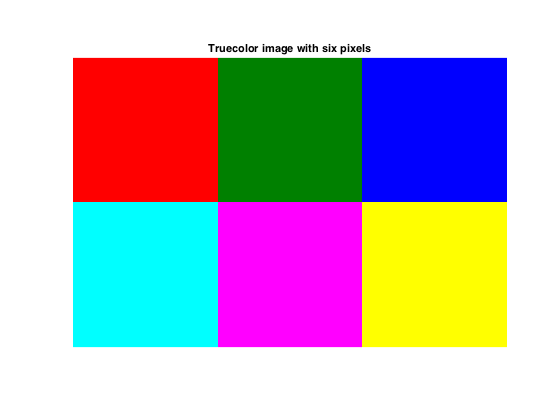With truecolor images, changing the colormap has no effect on the image colors displayed.

colormap(hot)
title('Changing the figure colormap does not affect the pixel colors')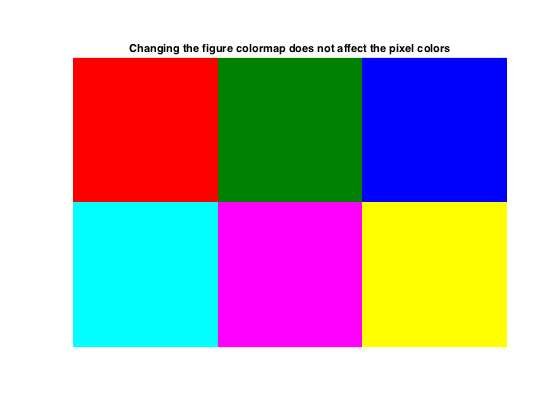#### Indexed images

If the image CData is two-dimensional, then the CData values are treated as lookup indices into the axes or the figure colormap. As an example, let's use an indexed image that ships with MATLAB, clown.mat (Ned's favorite).

s = load('clown')  % This the functional form of load.  This form returns
% a structure whose fields are the variables stored
% in the MAT-file.

s =

X: [200x320 double]
map: [81x3 double]
caption: [2x1 char]



The X and map variables stored in clown.mat are both necessary to display the image correctly. X contains the pixel values, and map contains the associated colormap.

To get the color of the (5,5) pixel, first see what X(5,5) is:

s.X(5,5)

ans =

61



Then use that value as a row index into the colormap matrix, map:

s.map(61,:)

ans =

0.9961    0.5781    0.1250



These are the same three color components (red, green, and blue) that are used for truecolor images. So the (5,5) pixel has a lot of red, some green, and a small amount of blue.

To display the image, pass both the CData and the colormap to imshow.

imshow(s.X,s.map)
title('Indexed image')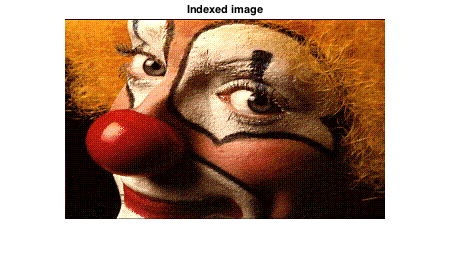Unlike truecolor images, indexed images are affected by changed in the figure's colormap.

colormap(cool)
title('Indexed image displays incorrectly if you use the wrong colormap')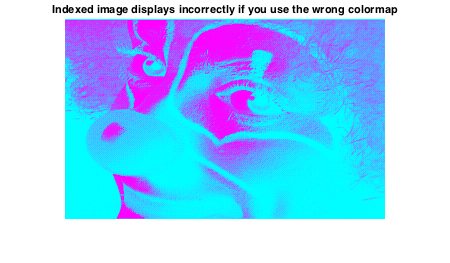In my previous post, I suggested that there might really be three pixel-color display models in MATLAB instead of two. The third display model is a variation of the indexed image model.

I'll talk about that next time.

Published with MATLAB® R2015b

|

### Comments

To leave a comment, please click here to sign in to your MathWorks Account or create a new one.